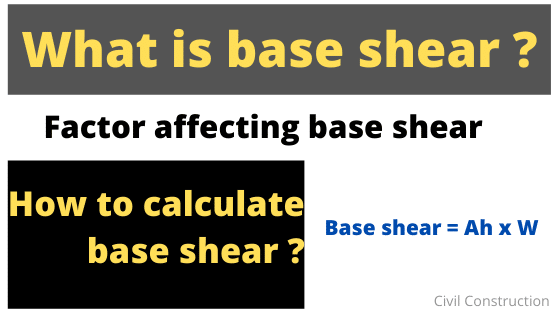Base shear is the estimation of maximum expected lateral force which will occur at the base of a structure due ground motion during the earthquake. Due to seismic activities, the ground start moving. Due to the movement of ground, lateral force is developed in opposite direction of motion. That developed lateral force due seismic motion at the base of the structure is called base shear.

When we design the building, the building will give reaction from zero to base shear value. We design the building for base shear value not for the intermediate value. The building is made such that it can take lateral load or load due to earthquake that is equal to base shear of the building. The lateral force beyond the base shear results in collapse of the building.

Base of structure:

Base of the structure is the level at which inertial forces generated in the building are considered to be transferred to the ground through the foundation. For buildings with basements, it is considered at the bottom most basement level. For building resting on,

1. Pile foundation; it is considered to be the top of pile cap
2. Raft; it is considered to be top of the raft
3. Footing ; it is considered to be at the top of the footings

For buildings with combined types of foundation, the base is considered as the bottom-most level of the bases the constituent individual foundation as per definition above.

Factor affecting base shear:

The calculation of base shear VB depend on following factors:

• Soil type at the site
• Proximity to potential source of seismic activities ( such as geological faults)
• Probability of significant seismic ground motion
• The level of ductility and over-strength associated with various structural configuration and total weight of the structure
• The natural period of vibration of the structure when subjected to dynamic loading

Method of analysis of seismic load:

The effect of seismic loads on the structures can be considered in two ways:

1. Equivalent static method, and
2. Dynamic analysis method

In this post, we discuss about equivalent static method only and dynamic analysis will be discussed in upcoming post. Equivalent static method can be used for the analysis of regular structure with approximate natural period Ta less than 0.4 s. This method is applicable for regular building with height less than 15m in seismic zone II.

The design base shear VB along any principle direction of the building shall be determined by:

VB = Ah W

where,

Ah = design horizontal acceleration coefficient value as per clause 6.4.2 of IS 1893 (part 1) : 2016

W = seismic weight of the building

The seismic weight of the building is taken as the sum of the seismic weights of all the floors. Seismic weight of each floor is its full dead load plus appropriate amount of imposed load as specified in table 10.The weight of the column and walls in any storey shall be approximately apportioned to the floors above and below the storey while computing the seismic weight of each floor

Any weight supported in between storey shall be distributed to floor above and bottom in inverse proportional to its distance from the floors.

Seismic acceleration coefficient Ah

The design horizontal seismic acceleration coefficient Ah for a structure shall be determined by the given expression as,

Ah=ZISa / 2Rg

Where,

Z = seismic zone factor given by IS 1893 (Part I): 2016 Table 3

I = Importance factor given in IS 1893 for the corresponding structures; when not specified, the minimum value of I shall be,

1.5 for critical and lifeline structure,

1.2 for business continuity structure and

1 for general purpose building

R = Response reduction factor for the corresponding building given by IS 1893 (Part I): 2016 Table 9

Sa/𝑔 = design acceleration coefficient for different soil types, normalized with peak ground movement acceleration, corresponding to approximate fundamental natural period Ta of structure. It shall be taken as that corresponding to 5 percent of damping, given by IS 1893 (part 1) : 2016

The approximate fundamental natural period Ta of oscillation, in second, shall be estimated as mentioned in IS 1893(part1): 2016 clause 7.6.2

Ta = 0.09h/√d [For building other than with RC structural wall or without any masonry infills]

Where, h = height of building in m

d = base dimension at the plinth level of the building along the direction of earthquake shaking considered, in m

The deign base shear VB computed from the above equation shall be distributed along the height of the building and in plan at each floor level as below;

iThe base shear is vertically distributed to different floor level – the design base shear VB computed above shall be distributed along the height of the building as per the following expression:

Qi = [Wi hi2 / ∑Wi hi2 ] VB

Where,

Qi = design lateral force at floor i;

Wi = seismic weight of floor i;

hi = ith floor height measured from base